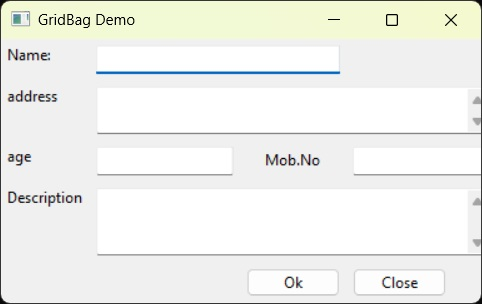# wxPython - GridBagSizer

GridBagSizer is a versatile sizer. It offers more enhancements than FlexiGridSizer. Child widget can be added to a specific cell within the grid. Furthermore, a child widget can occupy more than one cell horizontally and/or vertically. Hence, a static text and multiline text control in the same row can have different width and height.

Gridbag layout should be meticulously planned by deciding the position, span and the gap. wx.GridBagSizer class has only one constructor taking two arguments.

```Wx.GridBagSizer(vgap,hgap)
```

The most important method of GridBagsizer class is Add() which takes position as the mandatory argument. Span, alignment, border flags, and border size parameters are optional. If not explicitly used they assume default values.

```Wx.GridbagSizer().Add(control, pos, span, flags, border)
```

The following table lists the methods of GridBagSizer class −

S.N. Methods & Description
1

Adds given control at the specified position in the grid

2

GetItemPosition()

Returns the position of control in the grid

3

SetItemPosition()

Places a control at the specified position in the grid

4

GetItemSpan()

Returns row/column spanning of an item

5

SetItemSpan()

Spans the specified item over the number of rows/columns

The following code displays a form in which there are labels (StaticText) associated with textboxes (TexCtrl). TextCtrl objects are added with span parameter specified. Hence, the width of text boxes spans more than one column. Text box for name spans over two columns.

```tc = wx.TextCtrl(panel)
sizer.Add(tc, pos = (0, 1), span = (1, 2), flag = wx.EXPAND|wx.ALL, border = 5)
```

Textbox for address is a multiline text control spanning over three columns.

```tc1 = wx.TextCtrl(panel,style = wx.TE_MULTILINE)
sizer.Add(tc1, pos = (1,1), span = (1, 3), flag = wx.EXPAND|wx.ALL, border = 5)
```

The row containing multiline text control for description is set to be growable so that it expands vertically downwards, if the form is stretched.

```tc4 = wx.TextCtrl(panel,style = wx.TE_MULTILINE)
sizer.Add(tc4, pos = (3,1), span = (1,3), flag = wx.EXPAND|wx.ALL, border = 5)
```

Following is the complete code −

```import wx

class Example(wx.Frame):

def __init__(self, parent, title):
super(Example, self).__init__(parent, title = title)

self.InitUI()
self.Centre()
self.Show()

def InitUI(self):

panel = wx.Panel(self)
sizer = wx.GridBagSizer(0,0)

text = wx.StaticText(panel, label = "Name:")
sizer.Add(text, pos = (0, 0), flag = wx.ALL, border = 5)

tc = wx.TextCtrl(panel)
sizer.Add(tc, pos = (0, 1), span = (1, 2), flag = wx.EXPAND|wx.ALL, border = 5)

text1 = wx.StaticText(panel, label = "address")
sizer.Add(text1, pos = (1, 0), flag = wx.ALL, border = 5)

tc1 = wx.TextCtrl(panel,style = wx.TE_MULTILINE)
sizer.Add(tc1, pos = (1,1), span = (1, 3), flag = wx.EXPAND|wx.ALL, border = 5)

text2 = wx.StaticText(panel,label = "age")
sizer.Add(text2, pos = (2, 0), flag = wx.ALL, border = 5)

tc2 = wx.TextCtrl(panel)
sizer.Add(tc2, pos = (2,1), flag = wx.ALL, border = 5)

text3 = wx.StaticText(panel,label = "Mob.No")
sizer.Add(text3, pos = (2, 2), flag = wx.ALIGN_CENTER|wx.ALL, border = 5)

tc3 = wx.TextCtrl(panel)
sizer.Add(tc3, pos = (2,3),flag = wx.EXPAND|wx.ALL, border = 5)

text4 = wx.StaticText(panel, label = "Description")
sizer.Add(text4, pos = (3, 0), flag = wx.ALL, border = 5)

tc4 = wx.TextCtrl(panel,style =  wx.TE_MULTILINE)
sizer.Add(tc4, pos = (3,1), span = (1,3), flag = wx.EXPAND|wx.ALL, border = 5)

buttonOk = wx.Button(panel, label = "Ok")
buttonClose = wx.Button(panel, label = "Close" )

sizer.Add(buttonOk, pos = (4, 2),flag = wx.ALL, border = 5)
sizer.Add(buttonClose, pos = (4, 3), flag = wx.ALL, border = 5)

panel.SetSizerAndFit(sizer)

app = wx.App()
Example(None, title = 'GridBag Demo')
app.MainLoop()
```

The above code produces the following output −wxpython_layout_management.htm